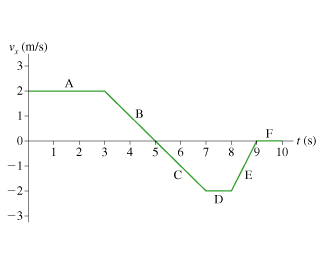# Velocity graph of an object speeding upDuring which segment(s) is the object speeding up?
During which segment(s) is the object slowing down?

I understand that the velocity is constant at A D E, and that it is moving in the positive right direction at segments A and B, but I'm confused at this part.

I originally thought that it is speeding up at E since the slope is positive and slowing down a B and C because the slope is negative but apparently those are incorrect.

Any help is appreciated!

Last edited:

DrClaude
Mentor
I understand that the velocity is zero at A and D

What does speeding up and slowing down mean? (Hint: What is the relation between speed and velocity?)

What does speeding up and slowing down mean? (Hint: What is the relation between speed and velocity?)

I meant constant. My fault. It is also constant at F.

And speed is ignorant of direction and velocity is direction aware. Im not sure how to tell if it speeding up or slowing down on a graph though

DrClaude
Mentor
And speed is ignorant of direction and velocity is direction aware.
Can you write this in a mathematical form?

Can you write this in a mathematical form?

V= dX/t

a= dv/t

d being delta or final minus initial

DrClaude
Mentor
Sorry if I'm not clear, but you need the mathematical relation between speed and velocity.

NascentOxygen
Staff Emeritus
During which segment(s) is the object speeding up?
I think you can interpret that as "where will the speedometer needle be seen to be climbing?"

I think you can interpret that as "where will the speedometer needle be seen to be climbing?"

Would B, C, and E be correct? Because the speedometer would be "revving" up at those points? I figured any time the graph looks like it is increasing is when it is speeding up but I think im incorrect.

NascentOxygen
Staff Emeritus
Would B, C, and E be correct? Because the speedometer would be "revving" up at those points? I figured any time the graph looks like it is increasing is when it is speeding up but I think im incorrect.
Through region B the vehicle is seen to be steadily progressing from 2m/s to 0m/s.

Think about the slope of the graph and what it represents. T

Also, how does it differ from the slope of a distance vs time graph?

Think about the slope of the graph and what it represents. T

Also, how does it differ from the slope of a distance vs time graph?

B and C have a negative slope, E has a positive slope, and A, D, and F have no slopes.

E is the only one that I can see speeding up because it is going from -2 to 0, but apparently that is wrong

DrClaude
Mentor
B and C have a negative slope
That's from the point of view of the velocity, for which the sign indicates the direction, and may not correspond to slowing down. That's why I keep asking about speed: if you can convert the graph from velocity to speed, then everything will become clearer.

E is the only one that I can see speeding up because it is going from -2 to 0, but apparently that is wrong
Imagine someone telling you: "I was going at 2 m/s to the left, then I was going at 0 m/s." Would you tell that person they were speeding up?

Heres my take on it:
A = constant speed forward ( t = 0 - 3 seconds )
B = slowing to a stop ( t = 3 - 5 seconds )
C = Speeding up in reverse ( t = 5 - 7 seconds )
D = constant speed in reverse ( t = 7 - 8 seconds )
E = slowing to a stop in reverse ( t = 8 - 9 seconds )
F = remaining stopped ( 9 - 10 seconds )

DrClaude
Mentor
Heres my take on it:
A = constant speed forward ( t = 0 - 3 seconds )
B = slowing to a stop ( t = 3 - 5 seconds )
C = Speeding up in reverse ( t = 5 - 7 seconds )
D = constant speed in reverse ( t = 7 - 8 seconds )
E = slowing to a stop in reverse ( t = 8 - 9 seconds )
F = remaining stopped ( 9 - 10 seconds )
That looks ok.

Note that what I was trying to get you to write was ##s = | v |##, speed is the absolute value of veloscity (in 1D). If you take the absolute value, then the only segment of the graph where you see an increase is C.

•Steelers72
That looks ok.

Note that what I was trying to get you to write was ##s = | v |##, speed is the absolute value of veloscity (in 1D). If you take the absolute value, then the only segment of the graph where you see an increase is C.

Thank you for the help. So if speed= absolute value of velocity, you just replace all the negative values of velocity with positive values for speed since speed is a quality that neglects direction. It all makes sense now. Thank you for the help!!

CWatters